# Analysis: Emissions boost factor from a temperature variation

## Generic temperature variation

Suppose that the temperature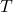is given by:

(1)where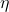is a temperature-variation with zero mean, so that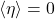. Then, the temperature-variation emissions boost factor,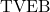or, will be given by:

(2)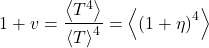(3)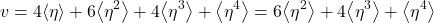Ifis small, i.e.,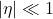, then this may be approximated by:

(4)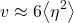From this, it also follows that, ifis small, then:

(5)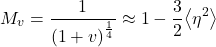## Contributions to emissions boost factor

Suppose we re-arrange one of the equations above to write:

(6)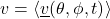where

(7)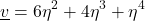(8)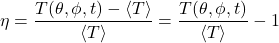This allows us to think oforas being made up of contributions from different times and places, contributions that are likely all positive (though I haven’t rigorously proved that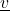must be positive). We might think ofas the local TVEB Contribution,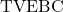.can alternatively be computed as:

(9)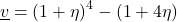Filling in the definition of, this becomes:

(10)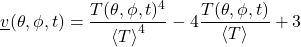Ifis small, this may be approximated as:

(11)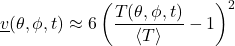The quantityoris interesting insofar as it offers a way of attributing contributions toorto different places and times (thoughis ultimately a non-local quantity).

This local version ofcould have been defined differently: adding or subtracting any multiple ofto the definition ofwould yield a quantity that averages to. So, it’s still unclear, as yet, if this way of definingis meaningful.

## Sinusoidal time variation

Suppose that the temperaturevaries as:

(12)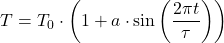where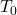and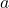are constants.

Ifis small compared to 1, this leads to the approximate result:

(13)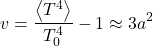(14)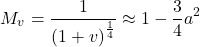As an example, suppose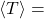288 K and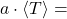5℃. Then this diurnal temperature variation would lead to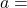0.017,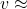9e-4 and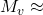1 – 2.3e-4, so that there the emissions boost would lead to a net temperature reduction (for the same average emissions) of about 0.065℃.

Studies show that global warming has been leading to night-time low temperatures increasing more than in day-time high temperatures. In other words,has been decreasing. The above result indicates that the reduction in the amplitude of diurnal temperature variation,, is not likely to contribute significantly to changes in the GMST (global mean surface temperature).